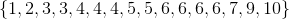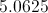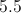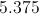# SSAT Middle Level Math : How to find mean

## Example Questions

← Previous 1 3 4 5 6 7 8 9 10

### Example Question #4 : Find Measures Of Center, Variability, And Patterns In Data: Ccss.Math.Content.6.Sp.B.5c

In a group of 4 books, the average number of pages is is 10.  Juan adds a book with 20 pages to the group.  What is the new average number of pages?Explanation:

If the average of 4 books was 10, then the total number of pages was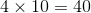. Adding in the 20 new pages, but now dividing by 5 you get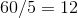.

### Example Question #1 : How To Find Mean

Give the mean of the data set (nearest tenth, if applicable):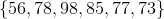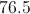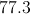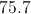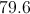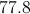Explanation:

Add the six elements and divide the sum by 6: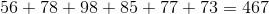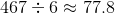### Example Question #2 : Find Mean

Find the mean of this set of numbers:

54, 41, 99, 120, 66.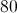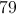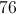Explanation: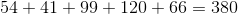Then, divide by the amount of numbers in the set: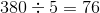### Example Question #3 : Find Mean

Give the mean of the following eight scores: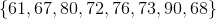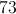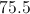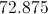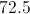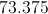Explanation:

Divide the sum of the scores by eight: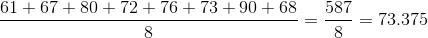### Example Question #1 : How To Find Mean

Give the mean of the following nine scores: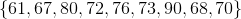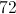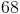Explanation:

Divide the sum of the scores by nine: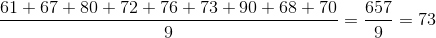### Example Question #1 : Median

Give the median of the following eight scores:Explanation:

Arrange the scores from least to greatest.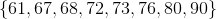There are an even number (eight) of scores, so the median is the arithmetic mean of the middle two scores, 72 and 73. This makes the median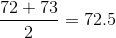### Example Question #9 : Find Measures Of Center, Variability, And Patterns In Data: Ccss.Math.Content.6.Sp.B.5c

The average age of 4 people is 12.  What will be the sum of their ages in 3 years?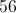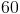Explanation:

The current sum of their ages is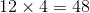.  Since all 4 people will gain 3 years in age, we have to add 12 to the current sum.

### Example Question #121 : Statistics & Probability

What is the mean of the values,,,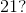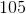Explanation:

The mean of a set of values can be calculated by adding the values together and dividing by the number of values: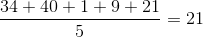### Example Question #1 : Mean

On the first four exams, Charley scored 92, 88, 76, and 74. Charley wants to have a test average of 85%.  What must Charley score on the fifth exam to average 85%?

85%

90%

95%

82%

100%

95%

Explanation:

The summation of the first four exams equals 330.  Using the equation for average, Charley needs to get a 95 on the last exam.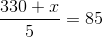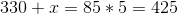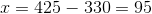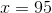### Example Question #1 : How To Find Mean

Give the mean of the following data set: